We’d like to understand how you use our websites in order to improve them. Register your interest.

# Asymptotics of solutions of the heat equation in cones and dihedra under minimal assumptions on the boundary

## Abstract

In the first part of the paper, the authors obtain the asymptotics of Green’s function of the first boundary value problem for the heat equation in an m-dimensional cone K. The second part deals with the first boundary value problem for the heat equation in the domain $K× R n − m$. Here the right-hand side f of the heat equation is assumed to be an element of a weighted $L p , q$-space. The authors describe the behavior of the solution near the $(n−m)$-dimensional edge of the domain.

## Introduction

The paper is concerned with the first boundary value problem for the heat equation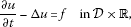(1)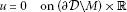(2)

in the domain

$D= { x = ( x ′ , x ″ ) : x ′ ∈ K , x ″ ∈ R n − m } ,$

where $K={ x ′ =( x 1 ,…, x m ): x ′ /| x ′ |∈Ω}$ is a cone in $R m$, $2≤m≤n$, Ω denotes a subdomain of the unit sphere, and $M={x=( x ′ , x ″ ): x ′ =0}$ is the $(n−m)$-dimensional edge of $D$. We are interested in the asymptotics of solutions in the class of the weighted Sobolev spaces $W p , q ; β 2 , 1 (D×R)$. Here the space $W p , q ; β 2 l , l (D×R)$ is defined for an arbitrary integer $l≥0$ and real $p>1$, $q>1$, β as the set of all function $u(x,t)$ on $D×R$ with the finite norm

$∥ u ∥ W p , q ; β 2 l , l ( D × R ) = ( ∫ R ( ∫ D ∑ | α | + 2 k ≤ 2 l | x ′ | p ( β − 2 l + 2 k + | α | ) | ∂ t k ∂ x α u ( x , t ) | p d x ) q / p d t ) 1 / q .$
(3)

In the case $l=0$, we write $W p , q ; β 0 , 0 = L p , q ; β$. If, moreover, $β=0$, then we write $L p , q ; 0 = L p , q$.

For the case of smooth boundary Ω (of class $C ∞$), the asymptotics of solutions was obtained in our previous paper . For the particular case $p=q=2$, $m=n$, we refer also to the paper  by Kozlov and Maz’ya, and for the case $p=q≠2$, $m=n=2$, to the paper  by de Coster and Nicaise. The goal of the present paper is to describe the asymptotics of solutions with a remainder in $W p , q ; β 2 , 1 (D×R)$ under minimal smoothness assumptions on the boundary. Throughout the paper, we assume that $∂Ω∈ C 1 , 1$.

The paper consists of two parts. The first part (Section 1) deals with the asymptotics of the Green function for the heat equation in the cone K. We obtain the same decomposition

$G ( x ′ , y ′ , t ) = ∑ λ j + < σ ∑ k = 0 m j ∂ t k c j ( y ′ , t ) | x ′ | λ j + + 2 k ϕ j ( ω x ) 4 k k ! ( σ j + k ) ( k ) + R σ ( x ′ , y ′ , t )$

as in [4, 5] (for the definition of $λ j +$, $ϕ j$, $m j$, $c j$ and $σ ( k )$, see Section 1.1). However, the proof in [4, 5] does not work if Ω is only of the class $C 1 , 1$. We give a new proof, which is completely different from that in [4, 5]. Our tools are estimates for solutions of the Dirichlet problem for the Laplace equation in a cone in weighted $L p$ Sobolev spaces and asymptotic formulas for solutions of this problem which were obtained in the papers [6, 7] by Maz’ya and Plamenevskiĭ. Moreover, we use the estimates of the Green function in the recent paper  by Kozlov and Nazarov. In contrast to the case $∂Ω∈ C ∞$, the estimates for the second order $x ′$- and $y ′$-derivatives of the remainder $R σ$ contain an additional factor $( | x ′ | − 1 d ( x ′ ) ) − ε$ with a negative exponent −ε. Here, $d( x ′ )$ is the distance from the boundary of ∂K.

In the second part of the paper (Section 2), we apply the results of Section 2 in order to obtain the asymptotics of solutions of the problem (1), (2) for $f∈ L p , q ; β (D×R)$. We show that, under a certain condition on β, there exists a solution of the form

$u(x,t)= ∑ λ j + < 2 − β − m / p ∑ k = 0 m j ( ∂ t − Δ x ″ ) k H j ( x , t ) 4 k k ! ( σ j + k ) ( k ) | x ′ | λ j + + 2 k ϕ j ( ω x )+w(x,t)$

with a remainder $w∈ W p , q ; β 2 , 1 (D×R)$. Here, $H j$ is an extension of the function

$h j ( x ″ , t ) = ∫ − ∞ t ∫ D c j ( y ′ , t − τ ) Φ ( x ″ , y ″ , t − τ ) f(y,τ)dydτ,$

Φ denotes the fundamental solution of the heat equation in $R n − m$. The proof of this result (Theorem 2.2) is essentially the same as in . However, the proofs of some lemmas in  have to be modified under our weaker assumptions on Ω.

At the end of the paper, we show that the extensions of the functions $h j$ can be defined as

$H j (x,t)=(E h j )(x,t)= ∫ 0 ∞ ∫ R n − m T(τ)R ( z ″ ) h j ( x ″ − r z ″ , t − r 2 τ ) d z ″ dτ,$

where T and R are certain smooth functions on $R +$ and $R n − m$, respectively (see the beginning of Section 3 for their definition). This extends the result of [, Corollary 4.5] to the case $p≠q$.

## 1 The Green function of the heat equation in a cone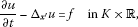(4)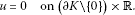(5)

Let $G( x ′ , y ′ ,t)$ be the Green function for the problem (4), (5). It is defined for every $y ′ ∈K$ as the solution of the problem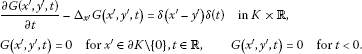Furthermore, $(1−ζ)G(⋅, y ′ ,⋅)∈ W 2 ; β 2 , 1 (K×R)$ if $λ 1 − <2−β−m/2< λ 1 +$ ($λ 1 ±$ are defined below), and ζ is a function in $C 0 ∞ (K×R)$ equal to one in a neighborhood of the point $( x ′ ,t)=( y ′ ,0)$. Here $W 2 , β 2 , 1 (K×R)$ is the space of all functions $u=u( x ′ ,t)$ on $K×R$ such that $| x ′ | β − 2 + 2 k + | α | ∂ t k ∂ x ′ α u∈ L 2 (K×R)$ for $2k+|α|≤2$. The goal of this section is to describe the behavior of the Green function for $| x ′ |< t$.

### 1.1 Asymptotics of Green’s function

Let ${ Λ j } j = 1 ∞$ be the nondecreasing sequence of eigenvalues of the Beltrami operator −δ on Ω (with the Dirichlet boundary condition) counted with their multiplicities, and let ${ ϕ j } j = 1 ∞$ be an orthonormal (in $L 2 (Ω)$) sequence of eigenfunctions corresponding to the eigenvalues $Λ j$. Furthermore, we define

$λ j ± = 2 − m 2 ± ( 1 − m / 2 ) 2 + Λ j and σ j = λ j + −1+ m 2 .$

This means that $λ j ±$ are the solutions of the quadratic equation $λ(m−2+λ)= Λ j$. Obviously, $λ j + >0$ and $λ j − <2−m$ for $j=1,2,…$ .

By [, Theorem 3],

$| ∂ t k ∂ x ′ α ∂ y ′ γ G ( x ′ , y ′ , t ) | ≤ c t − k − ( m + | α | + | γ | ) / 2 ( | x ′ | | x ′ | + t ) λ 1 + − | α | − ε ( | y ′ | | y ′ | + t ) λ 1 + − | γ | − ε × ( d ( x ′ ) | x ′ | ) − ε α ( d ( y ′ ) | y ′ | ) − ε γ exp ( − κ | x ′ − y ′ | 2 t )$
(6)

for $|α|≤2$, $|γ|≤2$. Here $d( x ′ )$ denotes the distance of the point $x ′$ from the boundary ∂K. Furthermore, $ε α$ is defined as zero for $|α|≤1$, while $ε α$ is an arbitrarily small positive real number if $|α|=2$. Actually, the estimate (6) is proved in  only for $k=0$, but for a more general class of operators, parabolic operators with discontinuous in time coefficients. If the coefficients in  do not depend on t, then one can use the same argument as in the proof of [, Theorem 3] when treating the derivatives along the edge of the domain $D=K× R n − m$. This argument shows that the k th derivative with respect to t will bring only an additional factor $t − k$ to the right-hand side of (6).

The following lemma will be applied in the proof of Lemma 1.2. Here and in the sequel, we use the notation $r=| x ′ |$ and $ω x = x ′ /| x ′ |$.

Lemma 1.1 Let $G( x ′ , y ′ ,t)$ be the Green function introduced above, and let $G j (r,ρ,t)$ denote the Green function of the initial-boundary value problem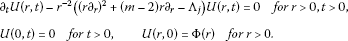Then

$∫ Ω G ( x ′ , y ′ , t ) ϕ j ( ω x )d ω x = | y ′ | 1 − m G j ( | x ′ | , | y ′ | , t ) ϕ j ( ω y ).$
(7)

Proof The solution of the problem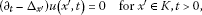(8)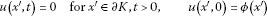(9)

is given by the formula

$u ( x ′ , t ) = ∫ K G ( x ′ , y ′ , t ) ϕ ( y ′ ) d y ′ .$

We define

$U j (r,t)= ∫ Ω u ( x ′ , t ) ϕ j ( ω x )d ω x .$

Then it follows from (8) and (9) that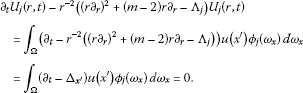Furthermore,

$U j (r,0)= Φ j (r) = def ∫ Ω ϕ ( x ′ ) ϕ j ( ω x )d ω x .$

Therefore,

$U j ( r , t ) = ∫ 0 ∞ G j ( r , ρ , t ) Φ j ( ρ ) d ρ = ∫ 0 ∞ ∫ Ω G j ( r , ρ , t ) ϕ j ( ω y ) ϕ ( y ′ ) d ω y d ρ = ∫ K G j ( r , | y ′ | , t ) ϕ j ( ω y ) ϕ ( y ′ ) | y ′ | 1 − m d y ′ .$

Comparing this with the formula

$U j (r,t)= ∫ Ω u ( x ′ , t ) ϕ j ( ω x )d ω x = ∫ K ∫ Ω G ( x ′ , y ′ , t ) ϕ j ( ω x )d ω x ϕ ( y ′ ) d y ′ ,$

we get (7). □

In the sequel, σ is an arbitrary real number satisfying the conditions

(10)

We define $G σ ( x ′ , y ′ ,t)=0$ for $σ< λ 1 +$, while

(11)

where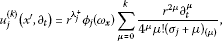(12)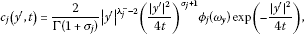(13)

and $m j =[ σ − λ j + 2 ]$. Here, we used the notation

We define $V p , β l (K)$ as the weighted Sobolev space with the norm

$∥ u ∥ V p , β l ( K ) = ( ∫ K ∑ | α | ≤ l r p ( β − l + | α | ) | ∂ x α u ( x ) | p d x ) 1 / p$

for $1 and integer $l≥0$.

Lemma 1.2 Suppose that σ is a real number such that $σ> λ 1 −$ and $(σ− λ j + )/2$ is not integer for $λ j + ≤σ$. Furthermore, let $1 and $β=2−σ−m/p$. Then

$G ( x ′ , y ′ , t ) = G σ ( x ′ , y ′ , t ) + R σ ( x ′ , y ′ , t ) ,$

where $∂ t k ∂ y ′ γ R σ (⋅, y ′ ,t)∈ V p , β 2 (K)$ for $y ′ ∈K$, $t>0$, $|γ|≤2$.

Proof We prove the lemma by induction in $m 1 =[(σ− λ 1 + )/2]$.

First, let $λ 1 − <σ< λ 1 +$. Then it follows from [, Corollary 4.1 and Theorem 4.2] (see also [, Theorem 3.2]) that $∂ t k ∂ y ′ γ G(⋅, y ′ ,t)∈ V p , β 2 (K)$ for all $y ′ ∈K$, $t>0$, $|γ|≤2$, where $β=2−σ−m/p$. Thus, the assertion of the lemma is true for $σ< λ 1 +$.

Suppose the assertion is proved for $σ< λ 1 + +2l$. Now let $λ 1 + +2l<σ< λ 1 + +2(l+1)$. We set $σ ′ =σ−2$ if $l>0$ and $σ ′ = λ 1 + −ε$ if $l=0$, where ε is a sufficiently small positive number. Then

By the induction hypothesis, we have

$G ( x ′ , y ′ , t ) = G σ ′ ( x ′ , y ′ , t ) + R σ ′ ( x ′ , y ′ , t ) ,$

where $G σ ′$ is given by (11) (with $σ ′$ instead of σ and $m j −1$ instead of $m j$), $∂ t k ∂ y ′ γ R σ ′ (⋅, y ′ ,t)∈ V p , β ′ 2 (K)$, $β ′ =2− σ ′ −m/p$. The coefficients $c j ( y ′ ,t)$ in $G σ ′$ are given by (13) and satisfy the equation $( ∂ t − Δ y ′ ) c j ( y ′ ,t)=0$. Therefore,

$( ∂ t − Δ y ′ ) R σ ′ ( x ′ , y ′ , t ) =0$

for $x ′ , y ′ ∈K$, $t>0$. Obviously, $G σ ′ (a x ′ ,a y ′ , a 2 t)= a − m G σ ′ ( x ′ , y ′ ,t)$ for $a>0$. Using the same equality for the Green function $G( x ′ , y ′ ,t)$, we obtain

Furthermore,

$Δ x ′ R σ ′ ( x ′ , y ′ , t ) = Δ x ′ G ( x ′ , y ′ , t ) − Δ x ′ G σ ′ ( x ′ , y ′ , t ) = ( ∂ t − Δ x ′ ) G σ ′ ( x ′ , y ′ , t ) + ∂ t R σ ′ ( x ′ , y ′ , t ) = ( ∂ t − Δ x ′ ) ∑ λ j + < σ ′ ∑ k = 0 m j − 1 ∂ t k c j ( y ′ , t ) 4 k k ! ( σ j + k ) ( k ) r λ j + + 2 k ϕ j ( ω x ) + ∂ t R σ ′ ( x ′ , y ′ , t ) .$

Using the formula

$Δ x ′ r λ j + + 2 k ϕ j (ω)=4k( σ j +k) r λ j + + 2 k − 2 ϕ j ( ω x ),$

we get

$Δ x ′ R σ ′ ( x ′ , y ′ , t ) = ∑ λ j + < σ ′ ∂ t m j c j ( y ′ , t ) r λ j + + 2 m j − 2 ϕ j ( ω x ) 4 m j − 1 ( m j − 1 ) ! ( σ j + m j − 1 ) ( m j − 1 ) + ∂ t R σ ′ ( x ′ , y ′ , t ) = Δ x ′ Σ ′ + ∂ t R σ ′ ( x ′ , y ′ , t ) ,$
(14)

where

$Σ ′ = ∑ λ j + < σ ′ ∂ t m j c j ( y ′ , t ) 4 m j m j ! ( σ j + m j ) ( m j ) r λ j + + 2 m j ϕ j ( ω x )$

($Σ ′ =0$ for $l=0$). Let χ be a smooth function with compact support on $[0,∞)$ such that $χ(r)=1$ for $r<1$. Using the notation $r=| x ′ |$, the function χ can be also considered as a function in K. Since $σ ′ < λ j + +2 m j <σ$ for $λ j + < σ ′$, we have $χ ∂ t k ∂ y ′ γ Σ ′ (⋅, y ′ ,t)∈ V p , β ′ 2 (K)$ and $(1−χ) ∂ t k ∂ y γ Σ ′ (⋅, y ′ ,t)∈ V p , β 2 (K)$ for all $y ′ ∈K$, $t>0$. Consequently, $∂ t k ∂ y ′ γ ( R σ ′ (⋅, y ′ ,t)−χ Σ ′ (⋅, y ′ ,t))∈ V p , β ′ 2 (K)$ and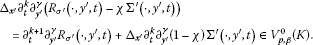Applying [, Theorem 4.2], we obtain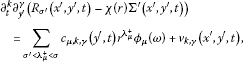(15)

where $v k , γ (⋅, y ′ ,t)∈ V p , β 2 (K)$. The coefficients $c μ , k , γ$ are given by the formula

$c μ , k , γ ( y ′ , t ) = ∫ K ∂ t k ∂ y ′ γ ( ∂ t R σ ′ ( x ′ , y ′ , t ) + Δ x ′ ( 1 − χ ) Σ ′ ( x ′ , y ′ , t ) ) v μ ( x ′ ) d x ′ ,$
(16)

where $v μ ( x ′ )=− 1 2 σ μ r λ μ − ϕ μ ( ω x )$. The integral in (16) is well defined, since

$∂ t k ∂ y ′ γ ( ∂ t R σ ′ ( ⋅ , y ′ , t ) + Δ x ′ ( 1 − χ ) Σ ′ ( ⋅ , y ′ , t ) ) ∈ V p , β 0 (K)∩ V p , β ′ 0 (K)$

and $v μ ∈ V p ′ , − β 0 (K)+ V p ′ , − β ′ 0 (K)$, $p ′ =p/(p−1)$, for $σ ′ < λ μ + <σ$. The remainder $v k , γ$ and the coefficients $c μ , k , γ$ in (15) satisfy the estimate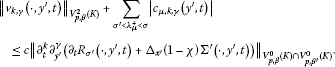(17)

Obviously, $c μ , k , γ ( y ′ ,t)= ∂ t k ∂ y ′ γ c μ ( y ′ ,t)= ∂ t k ∂ y ′ γ c μ , 0 , 0 ( y ′ ,t)$. This means that

$R σ ′ ( x ′ , y ′ , t ) −χ(r) Σ ′ ( x ′ , y ′ , t ) = ∑ σ ′ < λ μ + < σ c μ ( y ′ , t ) r λ μ + ϕ μ ( ω x )+v ( x ′ , y ′ , t ) ,$

where $∂ t k ∂ y ′ γ v(⋅, y ′ ,t)= v k , γ (⋅, y ′ ,t)∈ V p , β 2 (K)$. Consequently,

$R σ ′ ( x ′ , y ′ , t ) =Σ ( x ′ , y ′ , t ) + R σ ( x ′ , y ′ , t ) ,$
(18)

where

$Σ ( x ′ , y ′ , t ) = Σ ′ ( x ′ , y ′ , t ) + ∑ σ ′ < λ μ + < σ c μ ( y ′ , t ) r λ μ + ϕ μ ( ω x )= ∑ λ j + < σ ∂ t m j c j ( y ′ , t ) r λ j + + 2 m j ϕ j ( ω x ) 4 m j m j ! ( σ j + m j ) ( m j )$

and $R σ ( x ′ , y ′ ,t)=v( x ′ , y ′ ,t)+(χ−1) Σ ′ ( x ′ , y ′ ,t)$. Obviously, $∂ t k ∂ y ′ γ R σ (⋅, y ′ ,t)∈ V p , β 2 (K)$ for $|γ|≤2$. Using (18) and the equality

$G σ ′ ( x ′ , y ′ , t ) +Σ ( x ′ , y ′ , t ) = G σ ( x ′ , y ′ , t ) ,$

we conclude that

$G ( x ′ , y ′ , t ) = G σ ′ ( x ′ , y ′ , t ) + R σ ′ ( x ′ , y ′ , t ) = G σ ( x ′ , y ′ , t ) + R σ ( x ′ , y ′ , t ) .$

It remains to show that the coefficients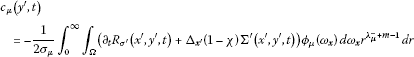(19)

in (15) have the form (13) for $σ ′ < λ μ + <σ$. First, note that

since $( ∂ t − Δ y ′ ) R σ ′ (x,y,t)=0$ and $( ∂ t − Δ y ′ ) Σ ′ (x,y,t)=0$.

Obviously, the functions $∂ t G σ ′ ( x ′ , y ′ ,t)$ and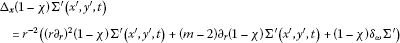contain only functions $ϕ j ( ω x )$ with $λ j + < σ ′$. Thus, the orthogonality of the functions $ϕ j$ implies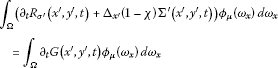(20)

for $λ μ + > σ ′$. Applying Lemma 1.1, we conclude that $c μ ( y ′ ,t)$ has the form

$c μ ( y ′ , t ) = ρ 1 − m ϕ μ ( ω y ) f μ (ρ,t),$
(21)

where $ρ=| y ′ |$. Since $R σ ′ (a x ′ ,a y ′ , a 2 t)= a − m R σ ′ ( x ′ , y ′ ,t)$ and $Σ ′ (a x ′ ,a y ′ , a 2 t)= a − m Σ ′ ( x ′ , y ′ ,t)$ for all $a>0$, it follows from (18) that

$∑ σ ′ < λ μ + < σ ( a λ μ + c μ ( a y ′ , a 2 t ) − a − m c μ ( y ′ , t ) ) r λ μ + ϕ μ ( ω x )= a − m R σ ( x ′ , y ′ , t ) − R σ ( a x ′ , a y ′ , a 2 t ) .$

The function on the right-hand side belongs to $V p , β 2 (K)$ for all $y ′ ∈K$, $t>0$, $a>0$, while the left-hand side belongs only to $V p , β 2 (K)$ if

$c μ ( a y ′ , a 2 t ) = a − m − λ μ + c μ ( y ′ , t ) .$

Combining the last equality with (21), we get the representation

$c μ ( y ′ , t ) = ρ − m − λ μ + ϕ μ ( ω y ) h μ ( ρ 2 4 t ) = ρ λ μ − − 2 ϕ μ ( ω y ) h μ ( ρ 2 4 t ) .$

Inserting this into the equation $( ∂ t − Δ y ′ ) c μ ( y ′ ,t)=0$, we obtain

$r 2 h μ ″ (r)+(r− σ μ −1)r h μ ′ (r)+( σ μ +1) h μ (r)=0.$

The substitution $h μ (r)= e − r r σ μ + 1 u(r)$ leads to the differential equation

$r 2 u ″ (r)+( σ μ +1−r)r u ′ (r)=0$

which has the solution

$u(r)= d 1 + d 2 ∫ r 1 s − σ μ − 1 e s ds$

with arbitrary constants $d 1$ and $d 2$. Consequently,

$c μ ( y ′ , t ) = ρ λ μ − − 2 ϕ μ ( ω y ) ( ρ 2 4 t ) σ μ + 1 exp ( − ρ 2 4 t ) ( d 1 + d 2 ∫ ρ 2 / ( 4 t ) 1 s − σ μ − 1 e s d s ) .$
(22)

Using (6) and (17), one gets the estimate

$| ∂ t k c μ ( y ′ , t ) | ≤ C k (t) ρ λ 1 + − ε$

with certain functions $C k$ for $ρ=|y|< t$. Thus, the constant $d 2$ in (22) must be zero. Integrating (19), we get

$∫ 0 ∞ c μ ( y ′ , t ) dt=− v μ ( y ′ ) = 1 2 σ μ ρ λ μ − ϕ μ ( ω y )$

by means of (20). Hence,

$d 1 ρ λ μ − − 2 ϕ μ ( ω y ) ∫ 0 ∞ ( ρ 2 4 t ) σ μ + 1 exp ( − ρ 2 4 t ) dt= 1 2 σ μ ρ λ μ − ϕ μ ( ω y ).$

The integral on the left-hand side is equal to $1 4 ρ 2 Γ( σ μ )$. Thus, we get $u(r)= d 1 =2/Γ( σ μ +1)$ and

$h μ (r)= 2 Γ ( σ μ + 1 ) r σ μ + 1 e − r .$

This means that the formula (13) is valid for the coefficients $c j$ if $σ ′ < λ j + <σ$. The proof of the lemma is complete. □

### 1.2 Point estimates for the remainder in the asymptotics of Green’s function

We are interested in point estimates for the remainder $R σ ( x ′ , y ′ ,t)$ in Lemma 1.2 in the case $| x ′ |< t$. For this, we need the following lemma.

Lemma 1.3 Suppose that $u∈ L p , β (K)$ and $d∇u∈ L p , β (K)$, where $p>m$. Then

$sup x ∈ K d ( x ′ ) m / p r ( x ) β | u ( x ′ ) | ≤c ( ∫ K r p β ( | d ( x ′ ) ∇ u ( x ′ ) | p + | u ( x ′ ) | p ) d x ′ ) 1 / p$

with a constant c independent of u.

Proof Let $x 0 ′$ be a point int K, and let $B 0$ be a ball centered at $x 0 ′$ with radius $d 0 /2=d( x 0 ′ )/2$. We introduce the new coordinates $y ′ = d 0 − 1 x ′$ and set $v( y ′ )=u( d 0 y ′ )=u( x ′ )$. Obviously, the point $y 0 ′ = d 0 − 1 x 0 ′$ has the distance 1 from ∂K. Hence,

$| v ( y 0 ′ ) | p ≤c ∫ | y ′ − y 0 ′ | < 1 / 2 ( | ∇ y ′ v ( y ′ ) | p + | v ( y ′ ) | p ) d y ′ .$

This implies

$| u ( x 0 ′ ) | p ≤c d 0 − m ∫ B 0 ( | d 0 ∇ x ′ u ( x ′ ) | p + | u ( x ′ ) | p ) d x ′ .$

Since $d 0 /2 and $r( x 0 ′ )/2 for $x ′ ∈ B 0$, we obtain

$d 0 m r ( x 0 ′ ) p β | u ( x 0 ′ ) | p ≤c ∫ B 0 r p β ( | d ( x ′ ) ∇ x ′ u ( x ′ ) | p + | u ( x ′ ) | p ) d x ′ .$

The result follows. □

Using the last two lemmas, we can prove the following theorem.

Theorem 1.1 Suppose that σ is a real number satisfying (10). Then

$G ( x ′ , y ′ , t ) = G σ ( x ′ , y ′ , t ) + R σ ( x ′ , y ′ , t ) ,$

where

$| ∂ t k ∂ x ′ α ∂ y ′ γ R σ ( x ′ , y ′ , t ) | ≤ c t − k − ( m + | α | + | γ | ) / 2 ( | x ′ | t ) σ − | α | ( | y ′ | | y ′ | + t ) λ 1 + − | γ | − ε × ( d ( x ′ ) | x ′ | ) − ε α ( d ( y ′ ) | y ′ | ) − ε γ exp ( − κ | y ′ | 2 t )$
(23)

for $| x ′ |< t$, $|α|≤2$, $|γ|≤2$. Here $ε α =0$ for $|α|≤1$, while $ε α$ is an arbitrarily small positive real number if $|α|=2$.

Proof Since $G σ = G σ + ε$ for small positive ε, we may assume, without loss of generality, that $(σ− λ j + )/2$ is not integer for $λ j + <σ$. We prove the theorem by induction in $m 1 =[(σ− λ 1 + )/2]$.

If $λ 1 − <σ< λ 1 +$, then the assertion of the theorem follows from [, Theorem 3]. Suppose that $λ 1 + +2l<σ< λ 1 + +2(l+1)$, $l≥0$, and that the theorem is proved for $σ< λ 1 + +2l$. We set $σ ′ =σ−2$ if $l>0$. In the case $l=0$, let $σ ′$ be an arbitrary real number satisfying the inequalities $λ 1 − < σ ′ < λ 1 +$ and $σ ′ ≥σ−2$. By the induction hypothesis, we have

$G ( x ′ , y ′ , t ) = G σ ′ ( x ′ , y ′ , t ) + R σ ′ ( x ′ , y ′ , t ) ,$

where $G σ ′$ is given by (11) (with $σ ′$ instead of σ and $m j −1$ instead of $m j$). Since $G σ ′ = G σ ′ + δ$ for sufficiently small δ, it follows from the induction hypothesis that

$| ∂ t k ∂ x ′ α ∂ y ′ γ R σ ′ ( x ′ , y ′ , t ) | ≤ c t − k − ( m + | α | + | γ | ) / 2 ( | x ′ | t ) σ ′ + δ − | α | ( | y ′ | | y ′ | + t ) λ 1 + − | γ | − ε × ( d ( x ′ ) | x ′ | ) − ε α ( d ( y ′ ) | y ′ | ) − ε γ exp ( − κ | y ′ | 2 t )$
(24)

for $| x ′ |<2 t$, $|α|≤2$, $|γ|≤2$. As was shown in the proof of Lemma 1.2, the remainder $R σ ′$ admits the decomposition

$R σ ′ ( x ′ , y ′ , t ) =Σ ( x ′ , y ′ , t ) + R σ ( x ′ , y ′ , t ) ,$

where

$Σ ( x ′ , y ′ , t ) = ∑ λ j + < σ r λ j + + 2 m j ϕ j ( ω x ) ∂ t m j c j ( y ′ , t ) 4 m j m j ! ( σ j + m j ) ( m j )$

and $∂ t k ∂ y ′ γ R σ (⋅, y ′ ,t)∈ V p , β 2 (K)$ for $t>0$, $y ′ ∈K$, $|γ|≤2$. Here $β=2−σ−m/p$. Furthermore (cf. (14)),

$Δ x ′ R σ ( x ′ , y ′ , t ) = Δ x ′ ( R σ ′ ( x ′ , y ′ , t ) − Σ ( x ′ , y ′ , t ) ) = Δ x ′ ( R σ ′ ( x ′ , y ′ , t ) − Σ ′ ( x ′ , y ′ , t ) ) = ∂ t R σ ′ ( x ′ , y ′ , t ) .$

Let χ be a smooth cut-off function on the interval $[0,∞)$, $χ=1$ in $[0,1)$ and $χ=0$ on $(2,∞)$. We define $χ 1 ( x ′ ,t)=χ( t − 1 / 2 | x ′ |)$ for $x ′ ∈K$, $t>0$. Then

$Δ x ′ ( χ 1 ( x ′ , t ) ∂ y ′ γ ∂ t k R σ ( x ′ , y ′ , t ) ) =f ( x ′ , y ′ , t ) ,$

where

$f= χ 1 ∂ y ′ γ ∂ t k + 1 R σ ′ +2 ∇ x ′ χ 1 ⋅ ∇ x ′ ∂ y ′ γ ∂ t k ( R σ ′ −Σ)+( Δ x ′ χ 1 ) ∂ y ′ γ ∂ t k ( R σ ′ −Σ).$

Thus, by [, Theorem 4.1], there exists a constant c such that

$∥ χ 1 ( ⋅ , t ) ∂ y ′ γ ∂ t k R σ ( ⋅ , y ′ , t ) ∥ V p , β 2 ( K ) ≤c ∥ f ( ⋅ , y ′ , t ) ∥ V p , β 0 ( K )$
(25)

for all $y ′ ∈K$, $t>0$, $|γ|≤2$. We estimate the norm of f. Using (24), we get

$∥ χ 1 ∂ t k + 1 ∂ y ′ γ R σ ′ ( ⋅ , y ′ , t ) ∥ V p , β 0 ( K ) ≤ c t − k − 1 − ( m + | γ | + σ ′ + δ ) / 2 ( | y ′ | | y ′ | + t ) λ 1 + − | γ | − ε exp ( − κ | y ′ | 2 t ) × ( d ( y ′ ) | y ′ | ) − ε γ ( ∫ | x ′ | < 2 t | x ′ | p ( β + σ ′ + δ ) d x ′ ) 1 / p .$

Here, $p(β+ σ ′ +δ)>−m$. Thus,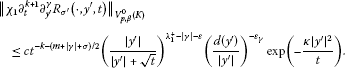Since $∇ x ′ χ 1$ vanishes outside the region $t <| x ′ |<2 t$ and $| ∂ x ′ α χ 1 ( x ′ ,t)|≤c t − | α | / 2$, the estimate (24) also yields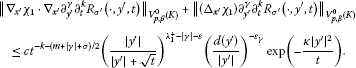Finally, it follows from the inequality

$| ∂ y ′ γ ∂ t k c μ ( y ′ , t ) | ≤c t − k − ( m + | γ | + λ μ + ) / 2 ( | y ′ | t ) λ μ + − | γ | exp ( − | y ′ | 2 6 t )$

that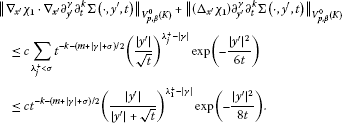Consequently, by (25),

$∥ χ 1 ( ⋅ , t ) ∂ y ′ γ ∂ t k R σ ( ⋅ , y ′ , t ) ∥ V p , β 2 ( K ) ≤ c t − k − ( m + | γ | + σ ) / 2 ( | y ′ | | y ′ | + t ) λ 1 + − | γ | − ε × ( d ( y ′ ) | y ′ | ) − ε γ exp ( − κ | y ′ | 2 t )$
(26)

with a positive constant κ. Applying the estimate

$∑ | α | ≤ 1 | x ′ | β − 2 + | α | + m / p | ∂ x ′ α χ 1 ( x ′ , t ) ∂ y ′ γ ∂ t k R σ ( x ′ , y ′ , t ) | ≤c ∥ χ 1 ∂ y ′ γ ∂ t k R σ ( ⋅ , y ′ , t ) ∥ V p , β 2 ( K )$

for $p>m$ (cf. [, Lemma 1.2.3]), we obtain (23) for $|α|≤1$.

It remains to prove the estimate (23) for $|α|=2$. Let $ρ( x ′ )$ be the “regularized distance” of the point $x ′$ to the boundary ∂K, i.e., ρ is a smooth function in K satisfying the inequalities

$c 1 d ( x ′ ) ≤ρ ( x ′ ) ≤ c 2 d ( x ′ )$

with positive constants $c 1$ and $c 2$ (cf. [, Chapter VI, § 2.1]). Moreover, ρ satisfies the inequality

$| ∂ x ′ α ρ ( x ′ ) | ≤cr ( x ′ ) 1 − | α | .$
(27)

We consider the function

$v ( x ′ , y ′ , t ) = χ 1 ( x ′ , t ) ρ ( x ′ ) ∂ x j ∂ y ′ γ ∂ t k R σ ( x ′ , y ′ , t )$

for $1≤j≤m$. It follows from the equation $Δ x ′ R σ = ∂ t R σ ′$ that

$Δ x ′ v= f 1 + f 2 + f 3 ,$

where $f 1 = χ 1 ρ ∂ x j ∂ y ′ γ ∂ t k + 1 R σ ′$, $f 2 =( Δ x ′ ( χ 1 ρ)) ∂ x j ∂ y ′ γ ∂ t k R σ$ and $f 3 =2 ∇ x ′ ( χ 1 ρ)⋅ ∇ x ′ ∂ x j ∂ y ′ γ ∂ t k R σ$. Using (24) and (27), we obtain

$∥ f 1 ( ⋅ , y ′ , t ) ∥ V p , β 0 ( K ) ≤c t − k − ( m + | γ | + σ ) / 2 ( | y ′ | | y ′ | + t ) λ 1 + − | γ | − ε ( d ( y ′ ) | y ′ | ) − ε γ exp ( − κ | y ′ | 2 t ) .$

Let $χ 2 ( x ′ ,t)=χ(| x ′ |/(2 t ))$. The inequalities $| Δ x ′ ( χ 1 ρ)|≤c r − 1$ and $| ∇ x ′ ( χ 1 ρ)|≤c$ yield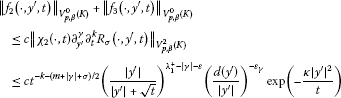(see (26)). Consequently by [, Theorem 4.1], the function $v= χ 1 ρ ∂ x j ∂ y ′ γ ∂ t k R σ$ satisfies the estimate

$∥ v ( ⋅ , y ′ , t ) ∥ V p , β 2 ( K ) ≤ c ∥ f 1 + f 2 + f 3 ∥ V p , β 0 ( K ) ≤ c t − k − ( m + | γ | + σ ) / 2 ( | y ′ | | y ′ | + t ) λ 1 + − | γ | − ε ( d ( y ′ ) | y ′ | ) − ε γ exp ( − κ | y ′ | 2 t ) .$

Applying Lemma 1.3 to the function $u( x ′ , y ′ ,t)= χ 1 ( x ′ ,t) ∂ x ′ α ∂ y ′ γ ∂ t k R σ ( x ′ , y ′ ,t)$ with an arbitrary multi-index α with length $|α|=2$, we get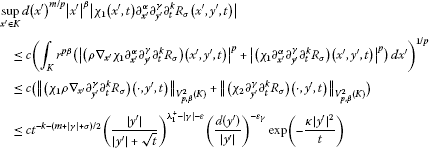for $|α|=2$, $|γ|≤2$, $p>m$. Since p can be chosen arbitrarily large, the estimate (23) holds in the case $|α|=2$. The proof is complete. □

## 2 Asymptotics of solutions of the problem in $D$

Now we consider the problem (1), (2) in the domain $D$. Throughout this section, it is assumed that $f∈ L p , q ; β (D×R)$, where p and β satisfy the inequalities

(28)

and q is an arbitrary real number >1. Let $G( x ′ , y ′ ,t)$ be the Green function of the problem (4), (5). Furthermore, let

$Φ ( x ″ , y ″ , t ) = ( 4 π t ) ( m − n ) / 2 exp ( − | x ″ − y ″ | 2 4 t )$

be the fundamental solution of the heat equation in $R n − m$. Then

$G(x,y,t)=G ( x ′ , y ′ , t ) Φ ( x ″ , y ″ , t )$

is the Green function of the problem (1), (2). We consider the solution

$u(x,t)= ∫ − ∞ t ∫ D G(x,y,t−τ)f(y,τ)dydτ$
(29)

of the problem (1), (2).

We again denote by $G σ ( x ′ , y ′ ,t)$ the function (11) introduced in Section 1. In the sequel, σ is an arbitrary real number such that

(30)

and

(31)

Then $G σ ( x ′ , y ′ ,t)= G 2 − β − m / p ( x ′ , y ′ ,t)$. Let χ be an infinitely differentiable function on $R + =(0,∞)$ equal to one on the interval $(0,1)$ and vanishing on $(2,∞)$. We define

$χ 1 ( x ′ , y ′ ) =χ ( | x ′ | | y ′ | ) , χ 2 ( x ′ , t , τ ) =χ ( | x ′ | t − τ ) .$

Obviously,

$u=Σ+v,$

where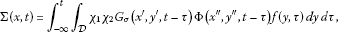(32)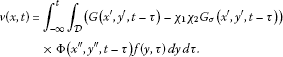(33)

We also consider the decomposition

$u= Σ ′ +w,$

where

$Σ ′ = ∑ λ j + < 2 − β − m / p u j ( m j ) ( x ′ , ∂ t − Δ x ″ ) H j (x,t)$
(34)

and

$H j (x,t)= ∫ − ∞ t ∫ D χ 1 ( x ′ , y ′ ) χ 2 ( x ′ , t , τ ) c j ( y ′ , t − τ ) Φ ( x ″ , y ″ , t − τ ) f(y,τ)dydτ$
(35)

is an extension of the function

$h j ( x ″ , t ) = ∫ − ∞ t ∫ D c j ( y ′ , t − τ ) Φ ( x ″ , y ″ , t − τ ) f(y,τ)dydτ$
(36)

with $c j$ defined by (13). Our goal is to show that both remainders v and w are elements of the space $W p , q ; β 2 , 1 (D×R)$. We start with the case $p=q$.

### 2.1 Estimates in weighted $L p$ Sobolev spaces

Let $W p , q ; β 2 l , l (D×R)$ be the weighted Sobolev space with the norm (3). Furthermore, let

$W p ; β 2 l , l (D×R)= W p , p ; β 2 l , l (D×R), L p ; β (D×R)= W p ; β 0 , 0 (D×R).$

In this subsection, we assume that $f∈ L p ; β (D×R)$, where p and β satisfy (28). First, we prove that $Σ− Σ ′ ∈ W p ; β 2 , 1 (D×R)$. This was shown in [, Corollary 2.3] for the case $∂Ω∈ C ∞$. In the case $∂Ω∈ C 1 , 1$, we must keep in mind that the second-order derivatives of the eigenfunctions $ϕ j$ must not be bounded. Then we have the estimate

$| ∂ x ′ α ϕ j ( ω x ) | ≤c | x ′ | − | α | ( d ( x ′ ) | x ′ | ) − ε α$
(37)

for $|α|≤2$, where $ε α =0$ for $|α|≤1$ and $ε α$ is an arbitrarily small positive real number if $|α|≤1$. However, this requires only a small modification of the proof in .

Lemma 2.1 Suppose that $f∈ L p , β (D×R)$. Then $∂ x α ∂ t k (Σ− Σ ′ )∈ L p ; β − 2 + | α | + 2 k (D×R)$ and

$∥ ∂ x α ∂ t k ( Σ − Σ ′ ) ∥ L p ; β − 2 + | α | + 2 k ( D × R ) ≤c ∥ f ∥ L p , β ( D × R )$

for $|α|≤2$ and all k.

Proof A simple calculation (see the proof of [, Corollary 1]) yields

$Σ − Σ ′ = − ∑ λ j + < σ ∫ − ∞ t ∫ D χ 1 ( x ′ , y ′ ) ( [ u j ( m j ) ( x ′ , ∂ t ) , χ 2 ] c j ( y ′ , t − τ ) ) × Φ ( x ″ , y ″ , t − τ ) f ( y , τ ) d y d τ ,$

where $[ u j ( m j ) ( x ′ , ∂ t ), χ 2 ]= u j ( m j ) ( x ′ , ∂ t ) χ 2 − χ 2 u j ( m j ) ( x ′ , ∂ t )$ denotes the commutator of $u j ( m j ) ( x ′ , ∂ t )$ and $χ 2$. Obviously, the inequalities

$| x ′ | ≤2 | y ′ | and t − τ ≤ | x ′ | ≤2 t − τ$

are satisfied on the support of the kernel

$K j (x,y,t,τ)= χ 1 ( x ′ , y ′ ) ( [ u j ( m j ) ( x ′ , ∂ t ) , χ 2 ] c j ( y ′ , t − τ ) ) Φ ( x ″ , y ″ , t − τ ) .$
(38)

Since, moreover, the eigenfunctions $ϕ j$ satisfy the inequality (37) for $|α|≤2$, we obtain

$| ∂ x α ∂ t k K j ( x , y , t , τ ) | ≤c ( t − τ ) − n / 2 ( d ( x ′ ) | x ′ | ) − ε | x ′ | − | α | − 2 k − σ | y ′ | σ exp ( − | y ′ | 2 + | x ″ − y ″ | 2 8 ( t − τ ) )$

for $|α|≤2$. Using Hölder’s inequality, we obtain

$| ∂ x α ∂ t k ( Σ − Σ ′ ) ( x , t ) | ≤c ( d ( x ′ ) | x ′ | ) − ε | x ′ | − | α | − 2 k − σ A 1 / p B 1 / p ′ ,$

where

$A= ∫ t − | x ′ | 2 t − | x ′ | 2 / 4 ∫ D ( t − τ ) − n / 2 | y ′ | p β | f ( y , τ ) | p exp ( − | y ′ | 2 + | x ″ − y ″ | 2 8 ( t − τ ) ) dydτ$

and

$B= ∫ t − | x ′ | 2 t − | x ′ | 2 / 4 ∫ D | y ′ | > | x ′ | / 2 ( t − τ ) − n / 2 | y ′ | p ′ ( σ − β ) exp ( − | y ′ | 2 + | x ″ − y ″ | 2 8 ( t − τ ) ) dydτ.$

The substitution $y ′ = z ′ t − τ$, $y ″ = x ″ + z ″ t − τ$ yields

$B ≤ c ∫ t − | x ′ | 2 t − | x ′ | 2 / 4 ( t − τ ) p ′ ( σ − β ) / 2 d τ ∫ | z ′ | > 1 / 2 | z ′ | p ′ ( σ − β ) exp ( − | z ′ | 2 8 ) d z ′ × ∫ R n − m exp ( − | z ″ | 2 8 ) d z ″ ,$

i.e., $B≤c | x ′ | p ′ ( σ − β ) + 2$. Consequently,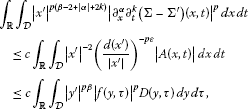where

$D ( y , τ ) = ∫ τ τ + | y ′ | 2 ∫ D t − τ < | x ′ | < 2 t − τ | x ′ | − 2 ( d ( x ′ ) | x ′ | ) − p ε ( t − τ ) − n / 2 × exp ( − | y ′ | 2 + | x ″ − y ″ | 2 8 ( t − τ ) ) d x d t .$

Substituting $x ′ = z ′ t − τ$ and $x ″ = y ″ + z ″ t − τ$, we obtain

$D(y,τ)= ∫ τ τ + | y ′ | 2 ( t − τ ) − 1 exp ( − | y ′ | 2 8 ( t − τ ) ) dt ∫ K 1 < | z ′ | < 2 | z ′ | − 2 ( d ( z ′ ) | z ′ | ) − p ε d z ′ .$

This means that $D(y,τ)$ is a constant. This proves the lemma. □

Next, we estimate the first-order x-derivatives of the remainder v. For this, we employ the following lemma (cf. [, Lemma A.1]).

Lemma 2.2 Let $K$ be the integral operator

$(Kf)(x,t)= ∫ − ∞ t ∫ R n K(x,y,t,τ)f(y,τ)dydτ$
(39)

with a kernel $K(x,y,t,τ)$ satisfying the estimate

$|K|≤c ( t − τ ) − ( n + 2 − r ) / 2 ( | x ′ | | x ′ | + t − τ ) a + r ( | y ′ | | y ′ | + t − τ ) b | x ′ | μ − r | y ′ | μ exp ( − κ | x − y | 2 t − τ ) ,$

where $κ>0$, $0, $a+b>−m$, $− m p −a<μ. Then $K$ is bounded on $L p ( R n ×R)$.

In the proof of the following assertion, we use another decomposition of the remainder v as in [, Lemma 2.4]. This allows us to apply directly the estimate in Theorem 1.1.

Lemma 2.3 Let p and β satisfy the condition (28). Furthermore, let v be the function (33), where $f∈ L p ; β (D×R)$, $1. Then $∂ x α v∈ L p ; β − 2 + | α | (D×R)$ for $|α|≤1$ and

$∑ | α | ≤ 1 ∥ ∂ x α v ∥ L p ; β − 2 + | α | ( D × R ) ≤c ∥ f ∥ L p ; β ( D × R )$

with a constant c independent of f. The same is true for the function w.

Proof Obviously,

$v= ∑ j = 1 3 ∫ − ∞ t ∫ D Ψ j (x,y,t,τ)f(y,τ)dydτ,$

where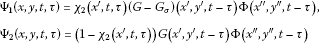and

$Ψ 3 (x,y,t,τ)= ( 1 − χ 1 ( x ′ , y ′ ) ) χ 2 ( x ′ , t , τ ) G σ ( x ′ , y ′ , t − τ ) Φ ( x ″ , y ″ , t − τ ) .$

We show that the integral operators with the kernels

$K j ( α ) (x,y,t,τ)= | x ′ | β − 2 + | α | | y ′ | − β ∂ x α Ψ j (x,y,t,τ)$

are bounded in $L p (D×R)$ for $j=1,2,3$ and $|α|≤1$. Using Theorem 1.1, we get

$| K 1 ( α ) ( x , y , t , τ ) | ≤ c | x ′ | β − 2 + | α | | y ′ | β ( t − τ ) − ( n + | α | ) / 2 ( | x ′ | t − τ ) σ − | α | ( | y ′ | | y ′ | + t − τ ) λ 1 + − ε × exp ( − κ | x − y | 2 t − τ ) ,$

where ε is an arbitrarily small positive number. Applying Lemma 2.2 with $r=2−|α|$, $μ=β$, $a=σ−2$, $b= λ 1 + −ε$, we conclude that the integral operator with the kernel $K 1 ( α ) (x,y,t,τ)$ is bounded in $L p (D×R)$ for $|α|≤1$.

Since $| x ′ |≤| x ′ |+ t − τ ≤2| x ′ |$ on the support of $K 2 ( α )$, the estimate (6) implies

$| K 2 ( α ) ( x , y , t , τ ) | ≤ c | x ′ | β − 2 + | α | | y ′ | β ( t − τ ) − ( n + | α | ) / 2 ( | x ′ | | x ′ | + t − τ ) a ( | y ′ | | y ′ | + t − τ ) λ 1 + − ε × exp ( − κ | x − y | 2 t − τ )$

with arbitrary real a. Thus, by Lemma 2.2, the integral operator with the kernel $K 2 (x,y,t,τ)$ is bounded in $L p (D×R)$ for $|α|≤1$.

We consider the kernel $K 3 ( α )$. Since $G σ ( x ′ , y ′ ,t)$ has the form

$G σ ( x ′ , y ′ , t ) = ∑ λ j + < σ ∑ k = 0 m j c j , k | x ′ | λ j + + 2 k | y ′ | λ j + ϕ j ( ω x ) ϕ j ( ω y ) ∂ t k t − λ j + − m / 2 exp ( − | y ′ | 2 4 t ) ,$

we get the representation

$K 3 ( α ) ( x ′ , y ′ , t , τ ) = ∑ λ j + < σ ∑ k = 0 m j K j , k (x,y,t,τ),$

where

$| K j , k ( x , y , t , τ ) | ≤c | x ′ | β − 2 + | α | | y ′ | β | x ′ | λ j + + 2 k − | α | | y ′ | λ j + ( t − τ ) − k − λ j + − n / 2 exp ( − κ | x − y | 2 t − τ ) .$

Here we used the fact that $| y ′ |≤| x ′ |≤2 t − τ$ on the support of the function $(1− χ 1 ) χ 2$. The inequalities $| y ′ |≤| x ′ |≤2 t − τ$ and $λ j + +2k≤σ$ imply

$| K j , k ( x , y , t , τ ) | ≤ c | x ′ | β − 2 + | α | | y ′ | β ( t − τ ) − ( n + | α | ) / 2 ( | x ′ | t − τ ) σ − | α | ( | y ′ | t − τ ) 2 λ 1 + − σ × exp ( − κ | x − y | 2 t − τ ) .$

It is no restriction to assume that $σ<2 λ 1 + +m−β−m/p$ in addition to (30) and (31). Therefore, we can apply Lemma 2.2 with $r=2−|α|$, $a=σ−2$ and $b=2 λ 1 + −σ$ to the integral operator with the kernel $K j , k$. It follows that the integral operator with the kernel $K 3 ( α ) (x,y,t,τ)$ is bounded in $L p (D×R)$ for $|α|≤1$. Consequently, the integral operator with the kernel

$K ( α ) (x,y,t,τ)= ∑ j = 1 3 K j ( α ) (x,y,t,τ)= | x ′ | β − 2 + | α | | y ′ | β ∑ j = 1 3 ∂ x α Ψ j (x,y,t,τ)$

is bounded in $L p (D×R)$ for $|α|≤1$. This proves the lemma. □

Furthermore, the assertions of [, Lemmas 2.5, 2.6, Theorem 2.7] are also valid if Ω is only of the class $C 1 , 1$. The proof under this weaker assumption on Ω does not require any modifications of the method in . We give here only the formulation of [, Theorem 2.7].

Theorem 2.1 Let $f∈ L p ; β (D×R)$, where p and β satisfy the condition (28). Then there exists a solution of the problem (1), (2) which has the form

$u= ∑ λ j + < 2 − β − m / p u j ( m j ) ( x ′ , ∂ t − Δ x ″ ) H j (x,t)+w,$

where $w∈ W p ; β 2 , 1 (D×R)$ and $u j ( k )$, $m j$, $H j$ are given by (12), (31) and (35), respectively. The functions $H j$ depend only on $| x ′ |$, $x ″$ and t and satisfy the estimates

$∥ ∂ t k ∂ x ″ γ H j ∥ L p ; β + λ j + + 2 k + | γ | − 2 ( D × R ) ≤ c k , γ ∥ f ∥ L p ; β ( D × R )$
(40)

for $2k+|γ|>2−β− λ j + −m/p$ and

$∥ ∂ t k ∂ x ′ α ∂ x ″ γ H j ∥ L p ; β + λ j + + 2 k + | α | + | γ | − 2 ( D × R ) ≤ c k , α , γ ∥ f ∥ L p ; β ( D × R )$
(41)

for all k, α, γ, $|α|≥1$.

### 2.2 Weighted $L p , q$ estimates for the remainder

We assume now that $f∈ L p , q ; β (D×R)$ and consider the decomposition

$u= Σ ′ +w$

of the solution (29), where $Σ ′$ is defined by (34). Our goal is to show that $w∈ W p , q ; β 2 , 1 (D×R)$ if p and β satisfy the condition (28). For the proof, we will use the next lemma which follows directly from [, Theorem 3.8].

Lemma 2.4 Suppose that $K$ is a linear operator on $L p ( R n ×R)$ satisfying the following conditions:

1. (i)

$∥ K h ∥ L p ( R n × R ) ≤ c 1 ∥ h ∥ L p ( R n × R )$ for all $h∈ L p ( R n ×R)$,

2. (ii)

$∫ | t − t 0 | > 2 δ ∥ ( K h ) ( ⋅ , t ) ∥ L p ( R n ) dt≤ c 2 ∫ R ∥ h ( ⋅ , t ) ∥ L p ( R n ) dt$ for all $δ>0$ and for all functions h with support in the layer $|t− t 0 |<δ$ such that $∫ R h(x,t)dt≡0$ .

Then the inequality

$∥ K h ∥ L p , q ( R n × R ) ≤c ∥ h ∥ L p , q ( R n × R )$

holds for arbitrary q, $1. Here the constant c depends only on $c 1$, $c 2$, p and q.

The condition (ii) of the last lemma can be verified in some cases by means of the following lemma (cf. [, Lemma 10]).

Lemma 2.5 Suppose that the kernel of the integral operator (39) satisfies the estimate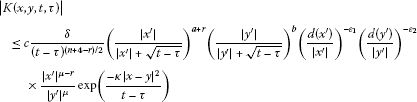for $t> t 0 +2δ$, $|τ− t 0 |≤δ$, where $κ>0$, $0≤r≤2$, $a+b>−m$, $− m p −a<μ, $0≤ ε 1 <1/p$, $0≤ ε 2 <1−1/p$. Then

$∫ t 0 + 2 δ ∞ ∥ ( K h ) ( ⋅ , t ) ∥ L p ( D ) dt≤c ∥ h ∥ L p , 1 ( D × R )$

for all $h∈ L p , 1 (D×R)$ with support in the layer $|t− t 0 |≤δ$. Here, the constant c is independent of $t 0$ and δ.

It is more easy to estimate the remainder $v=u−Σ$, where Σ is defined by (32). For this reason, we estimate the difference $Σ− Σ ′$ first.

Lemma 2.6 Let Σ and $Σ ′$ be the functions (32) and (34), respectively. If $f∈ L p , q ; β (D×R)$, then $∂ t k ∂ x α (Σ− Σ ′ )∈ L p , q ; β − 2 + 2 k + | α | (D×R)$ and

$∥ ∂ t k ∂ x α ( Σ − Σ ′ ) ∥ L p , q ; β − 2 + 2 k + | α | ( D × R ) ≤ c k , α ∥ f ∥ L p ; β ( D × R )$

for all k and α, $|α|≤2$. Here, the constants $c k , α$ are independent of f. In particular, $Σ− Σ ′ ∈ W p , q ; β 2 , 1 (D×R)$.

Proof We have

$Σ− Σ ′ =− ∑ λ j + < σ ∫ − ∞ t ∫ D K j (x,y,t,τ)f(y,τ)dydτ,$

where $K j$ is given by (38). Let $K j , k , α$ be the integral operator with the kernel

$K j , k , α (x,y,t,τ)= | x ′ | β − 2 + 2 k + | α | | y ′ | − β ∂ x α ∂ t k K j (x,y,t,τ),$

where $|α|≤2$. As was shown in the proof of Lemma 2.1, this operator is bounded in $L p (D×R)$. Now let h be a function in $L p , 1 (D×R)$ with support in the layer $|t− t 0 |≤δ$ satisfying the condition $∫ R h(x,t)dt≡0$. Then

$( K j , k , α h)(x,t)= ∫ − ∞ t ∫ D ( ∫ t 0 τ ∂ ∂ s K j , k , α ( x , y , t , s ) d s ) h(y,τ)dydτ.$

Analogously to the proof of Lemma 2.1, we obtain

$| ∂ ∂ s K j , k , α ( x , y , t , s ) | ≤c ( t − s ) − 1 − n / 2 ( d ( x ′ ) | x ′ | ) − ε | x ′ | β − σ − 2 | y ′ | β − σ exp ( − | x − y | 2 8 ( t − s ) )$
(42)

for $|α|≤2$. Since $| x ′ |≤| x ′ |+ t − s ≤2| x ′ |$ and $| y ′ |≤| y ′ |+ t − s ≤3| y ′ |$ on the support of $K j , k , α (x,y,t,s)$, we can append the factors

$( | x ′ | | x ′ | + t − s ) a and ( | y ′ | | y ′ | + t − s ) b$

with arbitrary exponents a and b on the right-hand side of (42). For $t> t 0 +2δ$ and $|τ−s|<|τ− t 0 |<δ$, we obviously have $(t−τ)/2. Consequently,

$| ∫ t 0 τ ∂ ∂ s K j , k , α ( x , y , t , s ) d s | ≤ c δ ( t − τ ) 1 + n / 2 ( d ( x ′ ) | x ′ | ) − ε | x ′ | β − σ − 2 | y ′ | β − σ × ( | x ′ | | x ′ | + t − τ ) a ( | y ′ | | y ′ | + t − τ ) b exp ( − | x − y | 2 8 ( t − s ) )$

for $t> t 0 +2δ$ and $|τ− t 0 |<δ$, where a and b are arbitrary real numbers and ε is an arbitrarily small positive real number. Hence, by Lemmas 2.4 and 2.5, the operator $K j , k , α$ is bounded in $L p , q (D×R)$ for $1.

We consider the operator $K ˜ j , k , α$ with the kernel

$K ˜ j , k , α (x,y,t,τ)= K j , k , α (y,x,−τ,−t)= ( − 1 ) k | y ′ | β − 2 + 2 k + | α | | x ′ | β ∂ τ k ∂ y α K j (y,x,−τ,−t).$

It follows from the boundedness of the operator $K j , k , α$ in $L p$ that $K ˜ j , k , α$ is bounded in $L p ′ (D×R)$, $p ′ =p/(p−1)$. Furthermore, one can check that

$| ∫ t 0 τ ∂ ∂ s K ˜ j , k , α ( x , y , t , s ) d s | ≤ c δ ( t − τ ) 1 + n / 2 ( d ( y ′ ) | y ′ | ) − ε | x ′ | σ − β | y ′ | σ − β + 2 × ( | x ′ | | x ′ | + t − τ ) a ( | y ′ | | y ′ | + t − τ ) b exp ( − | x − y | 2 8 ( t − s ) )$

with arbitrary a and b. Thus, as in the first part of the proof, we conclude that $K ˜ j , k , α$ (and therefore also the adjoint operator of $K j , k , α$) is bounded in $L p ′ , q ′ (D×R)$ for $1< q ′ < p ′$. This means that $K j , k , α$ is bounded in $L p , q (D×R)$ for all $p,q>1$. The lemma is proved. □

By means of Lemma 2.5, it is also possible to prove the assertion of [, Theorem 3.7] under the weaker assumption on Ω of the present paper.

Theorem 2.2 Let $f∈ L p , q ; β (D×R)$, where p and β satisfy the condition (28) and q is an arbitrary real number, $1. Then there exists a solution of the problem (1), (2) which has the form

$u= ∑ λ j + < 2 − β − m / p u j ( m j ) ( x ′ , ∂ t − Δ x ″ ) H j (x,t)+w,$

where $u j ( m j )$, $H j$ are given by (12) and (35), respectively, and $w∈ W p , q ; β 2 , 1 (D×R)$. The functions $H j$ are extensions of the functions (36) depending only on $| x ′ |$, $x ″$ and t and satisfy the estimate

$∥ ∂ t k ∂ x ′ α ∂ x ″ γ H j ∥ L p , q ; β + λ j + + 2 k + | α | + | γ | − 2 ( D × R ) ≤ c k , α , γ ∥ f ∥ L p , q ; β ( D × R )$
(43)

for all $k,α,γ$ such that $|α|≥1$ or $2k+|γ|>2−β− λ j + −m/p$.

Proof We have to show that the integral operator $K ( k , α )$ with the kernel

$K ( k , α ) (x,y,t,τ)= | x ′ | β − 2 + 2 k + | α | | y ′ | β ∂ t k ∂ x α (G− χ 1 χ 2 G σ ) ( x ′ , y ′ , t − τ ) Φ ( x ″ , y ″ , t − τ )$

is bounded in $L p , q (D×R)$ for $2k+|α|≤2$. For $p=q$ this is true by Theorem 2.1. Let $Ψ 1$, $Ψ 2$, and $Ψ 3$ be the same functions as in the proof of Lemma 2.3 and let

$K j ( k , α ) (x,y,t,τ)= | x ′ | β − 2 + 2 k + | α | | y ′ | − β ∂ x α ∂ t k Ψ j (x,y,t,τ).$

Then $K ( k , α ) = K 1 ( k , α ) + K 2 ( k , α ) + K 3 ( k , α )$. We show that the operators $K j ( k , α )$ satisfy the condition (ii) of Lemma 2.4. Let h be a function in $L p , 1 (D×R)$ with support in the layer $|t− t 0 |≤δ$ satisfying the condition $∫ R h(x,t)dt=0$ for all x. Then

$( K j ( k , α ) h ) (x,t)= ∫ − ∞ t ∫ D ( ∫ t 0 τ ∂ ∂ s K j ( k , α ) ( x , y , t , s ) d s ) h(y,τ)dydτ.$

Using Theorem 1.1, we get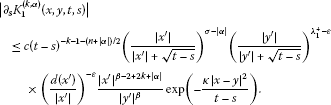Thus,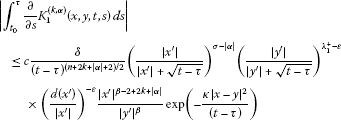for $t> t 0 +2δ$ and $|τ− t 0 |<δ$. Applying Lemma 2.5 with $r=2−2k−|α|$, $a=σ+2k−2$ and $b= λ 1 + −ε$, we conclude that

$∫ t 0 + 2 δ ∞ ∥ ( K j ( k , α ) h ) ( ⋅ , t ) ∥ L p ( D ) dt≤c ∥ h ∥ L p , 1 ( D × R )$
(44)

for $j=1$ and $2k+|α|≤2$. Analogously, the estimate (6) yields

$| ∫ t 0 τ ∂ ∂ s K 2 ( k , α ) ( x , y , t , s ) d s | ≤ c δ ( t − τ ) ( n + 2 k + | α | + 2 ) / 2 ( | x ′ | | x ′ | + t − τ ) a ( | y ′ | | y ′ | + t − τ ) λ 1 + − ε × ( d ( x ′ ) | x ′ | ) − ε | x ′ | β − 2 + 2 k + | α | | y ′ | β exp ( − κ | x − y | 2 ( t − τ ) )$

for $t> t 0 +2δ$ and $|τ− t 0 |<δ$, where a is an arbitrary real number. Here, we used the fact that $| x ′ |≤| x ′ |+ t − τ ≤2| x ′ |$ on the support of $K 2 ( k , α )$. Thus, by Lemma 2.5, the inequality (44) holds for $j=2$ and $2k+|α|≤2$.

Analogously to the estimation of the kernel $K 3 ( α )$ in the proof of Lemma 2.3, we obtain the estimate

$| ∫ t 0 τ ∂ ∂ s K 3 ( k , α ) ( x , y , t , s ) d s | ≤ c δ ( t − τ ) ( n + 2 + 2 k + | α | ) / 2 ( | x ′ | t − τ ) σ − | α | ( | y ′ | t − τ ) 2 λ 1 + − σ × ( d ( x ′ ) | x ′ | ) − ε | x ′ | β − 2 + 2 k + | α | | y ′ | β exp ( − κ | x − y | 2 t − τ )$

by means of (37). We may assume, without loss of generality, that $σ<2 λ 1 + +m−β−m/p$ in addition to (30) and (31). Then we conclude from Lemma 2.5 that (44) is valid for $j=3$ and $2k+|α|≤2$. Hence, by Lemma 2.4, the operator $K ( k , α )$ is bounded in $L p , q (D×R)$ for $1 if $2k+|α|≤2$.

In order to prove this for $q>p$, we consider the adjoint operator. Let $K ˜ ( k , α )$ and $K ˜ j ( k , α )$ be the integral operators with the kernels

$K ˜ ( k , α ) (x,y,t,τ)= K ( k , α ) (y,x,−τ,−t)and K ˜ j ( k , α ) (x,y,t,τ)= K j ( k , α ) (y,x,−τ,−t),$

respectively. From the boundedness of $K ( k , α )$ in $L p (D×R)$ it follows that $K ˜ ( k , α )$ is bounded in $L p ′ (D×R)$, $p ′ =p/(p−1)$. We show that

$∫ | t − t 0 | > 2 δ ∥ ( K ˜ j ( k , α ) h ) ( ⋅ , t ) ∥ L p ( D ) dt≤ c 2 ∫ R ∥ h ( ⋅ , t ) ∥ L p ( D ) dt$
(45)

for all $δ>0$, $j=1,2,3$ and for all functions h with support in the layer $|t− t 0 |<δ$ such that $∫ R h(⋅,t)dt=0$. Let h be such a function. Then

$( K ˜ j ( k , α ) h ) (x,t)= ∫ − ∞ t ∫ D ( ∫ t 0 τ ∂ ∂ s K ˜ j ( k , α ) ( x , y , t , s ) d s ) h(y,τ)dydτ.$

By means of 1.1, we obtain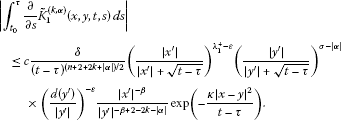Analogously, the estimate (6) implies

$| ∫ t 0 τ ∂ ∂ s K ˜ 2 ( k , α ) ( x , y , t , s ) d s | ≤ c δ ( t − τ ) ( n + 2 + 2 k + | α | ) / 2 ( | x ′ | | x ′ | + t − τ ) λ 1 + − ε ( | y ′ | | y ′ | + t − τ ) a × ( d ( y ′ ) | y ′ | ) − ε | x ′ | − β | y ′ | − β + 2 − 2 k − | α | exp ( − κ | x − y | 2 t − τ ) ,$

where a is an arbitrary real number, since $| y ′ |≤| y ′ |+ t − τ ≤2| y ′ |$ on the support of the function $K ˜ 2 ( k , α ) (x,y,t,τ)$. Applying Lemma 2.5, we obtain (45) for $2k+|α|≤2$ and $j≤2$. Using the representation for $G σ$, the estimate (37), and the fact that $| x ′ |≤| y ′ |≤2 t − τ$ on the support of $K ˜ 3 ( k , α ) (x,y,t,τ)$, we obtain

$| ∫ t 0 τ ∂ ∂ s K ˜ 3 ( k , α ) ( x , y , t , s ) d s | ≤ c δ ( t − τ ) ( n + 2 + 2 k ) / 2 ( | x ′ | | x ′ | + t − τ ) 2 λ 1 + − σ ( | y ′ | | y ′ | + t − τ ) σ × ( d ( y ′ ) | y ′ | ) − ε | x ′ | − β | y ′ | − β + 2 − 2 k exp ( − κ | x − y | 2 t − τ ) .$

We may assume again that $σ<2 λ 1 + +m−β−m/p$ in addition to (30) and (31). Then it follows from Lemma 2.5 that (45) is valid for $j=3$ and $2k+|α|≤2$. Therefore, by Lemma 2.4, the operator $K ˜ ( k , α )$ is bounded in $L p ′ , q ′ (D×R)$ for $1< q ′ < p ′$ if $2k+|α|≤2$. This means that $K ( k , α )$ is bounded in $L p , q (D×R)$ for all q if $2k+|α|≤2$. The proof of the theorem is complete. □

## 3 Another representation for the coefficients

As was proved [, Lemma 4.1], the functions $H j$ in Theorem 2.1 can be replaced by other extensions $H ˜ j$ of the functions $h j ( x ″ ,t)$ provided these extensions also satisfy the conditions (40) and (41). Note that the proof of this assertion in  is also correct under our assumptions on the boundary of Ω. Moreover, it was proved in [, Lemma 4.4], for the particular case $p=q$, that the extension

$H ˜ j (x,t)=(E h j )(x,t)= ∫ 0 ∞ ∫ R n − m T(τ)R ( z ″ ) h j ( x ″ − r z ″ , t − r 2 τ ) d z ″ dτ$

satisfies the conditions (40) and (41). Here $T(τ)$ is a smooth function with support in $[0,∞)$ satisfying the conditions

with certain positive constants $c k , M$, κ and

Furthermore, R is a smooth function with support on the cube $[ 0 , ( n − m ) − 1 / 2 ] n − m$ having the form

$R ( x ″ ) =R( x m + 1 ,…, x n )= ∏ j = m + 1 n ψ( x j ),$

where

(46)

with a sufficiently large integer $N 0$.

We extend the result of [, Lemma 4.4] to the case $q≠p$. First, note that $E h j = K j f$, where $K j$ is the integral operator

$( K j f)(x,t)= ∫ − ∞ t ∫ D K j (x,y,t−τ)f(y,τ)dydτ$

with the kernel

$K j (x,y,t)= r m − n − 2 ∫ 0 t ∫ R n − m T ( t − s r 2 ) R ( x ″ − z ″ r ) c j ( y ′ , s ) Φ ( y ″ , z ″ , s ) d z ″ ds.$

Our goal is to show that the operator

$L p , q ; β (D×R)∋f→ ∂ t k ∂ x ′ α ∂ x ″ γ K j f∈ L p , q ; β + λ j + + 2 k + | α | + | γ | − 2 (D×R)$

is bounded if $|α|≥1$ or $2k+|γ|>2−β− λ j + −m/p$. Since the function $(x,t)→( K j f)(x,t)$ depends only on the variables $r=| x ′ |$, $x ″$, and t, it suffices to prove that the operator

$L p , q ; β (D×R)∋f→ ∂ t k ∂ r l ∂ x ″ γ$